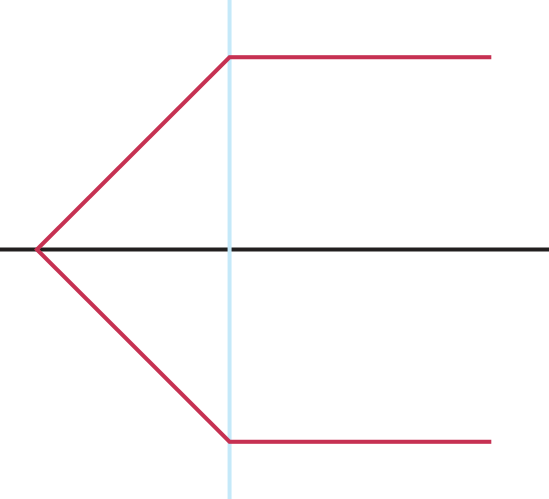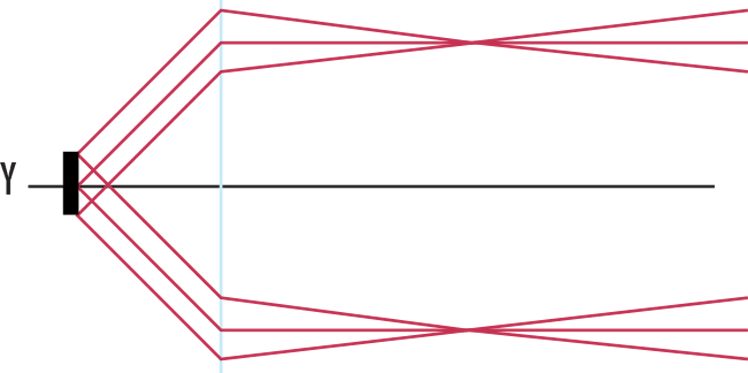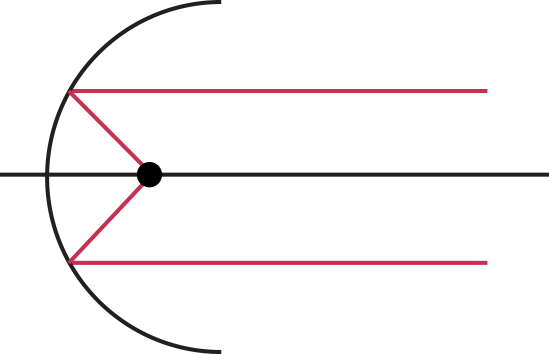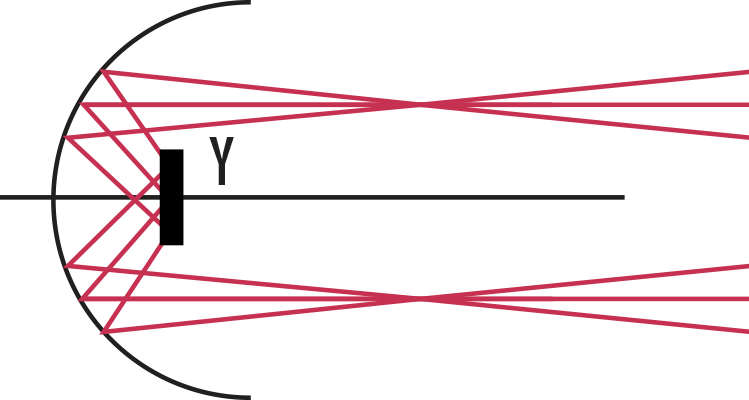# Considerations in Collimation

A collimated beam of light is defined when every ray within the beam is parallel to every other ray. To produce collimated light you can either place an infinitesimally small source exactly one focal length away from an optical system with a positive focal length or you can observe the point source from infinitely far away. In the real world, neither of these scenarios are possible. In addition, diffraction theory tells us that even if one of these scenarios were met, there would still be some divergence.

To minimize divergence of a collimated beam two factors must be balanced: focal length of the collimating system and size of the light source. Equation 1 approximates the divergence of a collimated beam:

(1)$$\text{Divergence} \approx \frac{\text{Size of Source}}{\text{Focal Length of Collimating System}}$$

To achieve ideal collimation, the size of the illumination source must be minimized or the focal length of the collimating system must be increased. Note that as you increase the focal length of the system, the system must be physically further away from the source. This means that less light will be captured by the focusing system and overall power in the resultant beam is decreased.

Some level of divergence will always be present when collimating light. In the illustration below, both systems produce collimated light, however, Figures 2 and 4 have more divergence compared to Figures 1 and 3 due to the sources being larger. Figures 2 and 4 can be thought of as a collection of closely spaced ideal point sources. Individually, each point creates a ray bundle of parallel rays, but as a collection the series of “point sources” create a beam with some divergence. The divergence exists because, as the size of the source increases, the source’s distance from the optical axis increases, and thus the resultant ray bundle’s angle increases with respect to the optical axis.##### Figure 1: Ideal Point Source with a Refractive Collimator##### Figure 2: Real Source with Height y and a Refractive Collimator##### Figure 3: Ideal Point Source with a Reflective Collimator